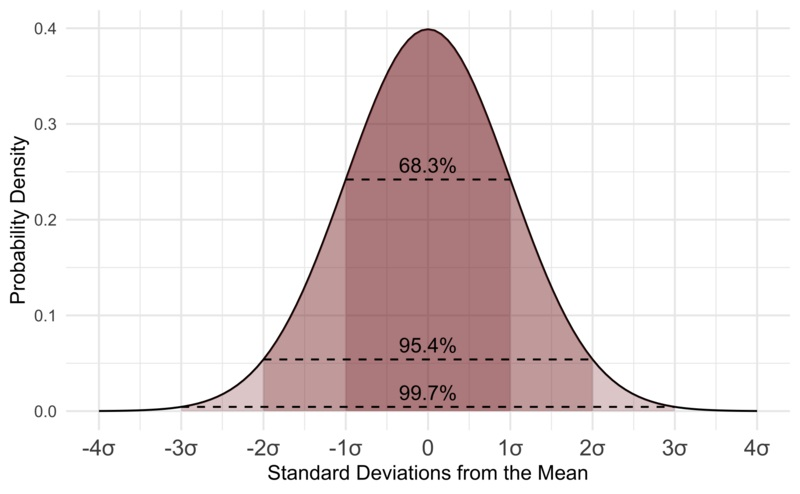# ML Mathematics

The main branches of Mathematics involved in Machine Learning are:

• Linear Functions
• Linear Graphics
• Linear Algebra
• Probability
• Statistics

## Machine Learning = Mathematics

Behind every ML success there is Mathematics.

All ML models are constructed using solutions and ideas from math.

The purpose of ML is to create models for understanding thinking.

If you want an ML career:

• Data Scientist
• Machine Learning Engineer
• Robot Scientist
• Data Analyst
• Natural Language Expert
• Deep Learning Scientist

You should focus on the mathematic concepts described here.

## Linear Functions

• Linear means straight
• A linear function is a straight line
• A linear graph represents a linear function

## Graphics

• Graphics plays an important role in Math
• Graphics plays an important role in Statistics
• Graphics plays an important role in Machine Learning

## Linear Algebra

Linear algebra is the bedrock of data science.

Knowing linear algebra boosts your ability to understand data science algorithms.

ScalarVector(s)
1
 1 2 3

 1 2 3

MatrixTensor
 1 2 3 4 5 6

 1 2 3 4 5 6

 4 5 6 1 2 3

## Probability

Probability is how likely something is to occur, or how likely something is true.

I have 6 balls in a bag: 3 reds, 2 are green, and 1 is blue.

Blindfolded. What is the probability that I pick a green one?

Number of ways it can happen are 2 (there are 2 greens).

Number of outcomes are 6 (there are 6 balls).

The probability is 2 out of 6: 2/6 = 0.333333...

Probability = Ways / Outcomes

## Statistics

Statistics is about how to collect, analyze, interpret, and present data.

Statistics works with questions like:

• What is the most Common?
• What is the most Expected?
• What is the most Normal?